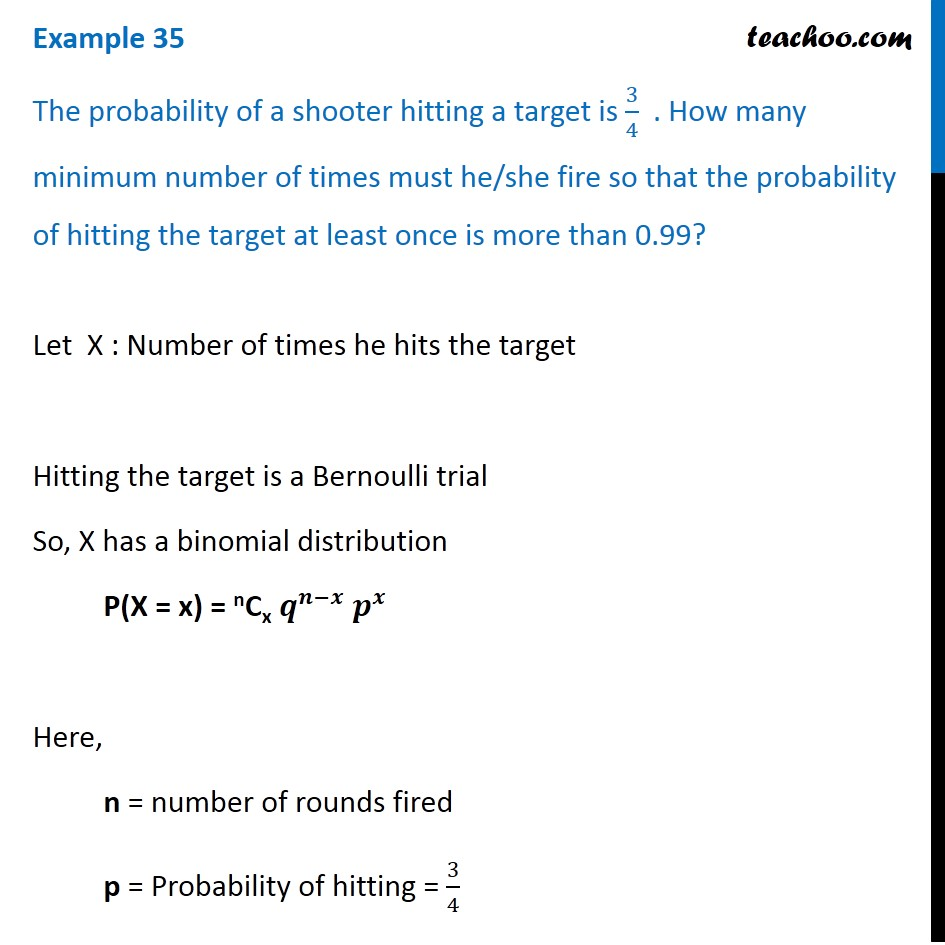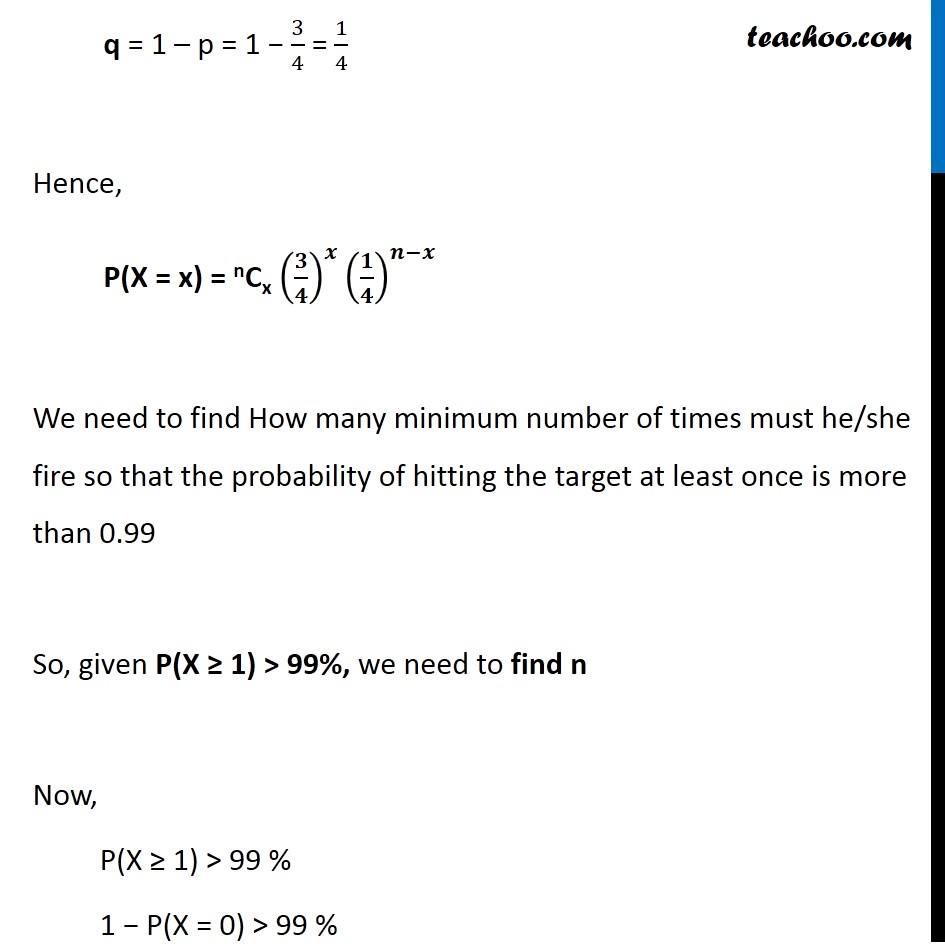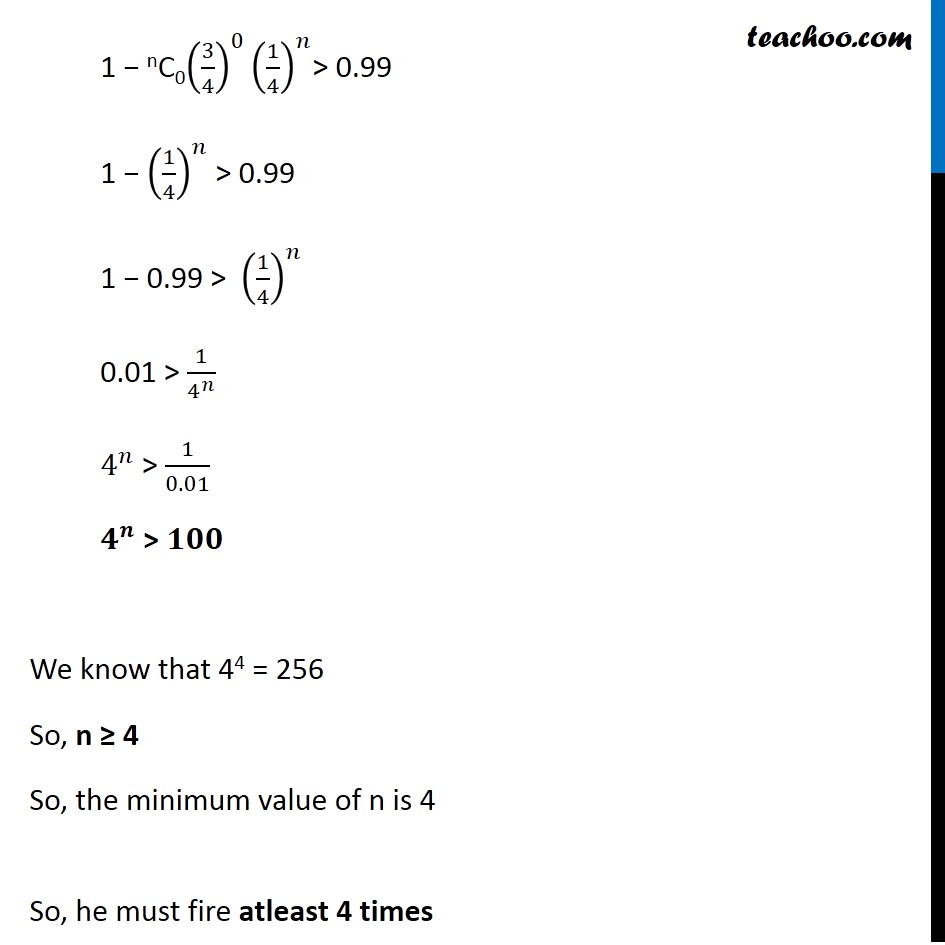Examples

Chapter 13 Class 12 Probability
Serial order wiseLearn in your speed, with individual attention - Teachoo Maths 1-on-1 Class

### Transcript

Question 13 The probability of a shooter hitting a target is 3/4 . How many minimum number of times must he/she fire so that the probability of hitting the target at least once is more than 0.99?Let X : Number of times he hits the target Hitting the target is a Bernoulli trial So, X has a binomial distribution P(X = x) = nCx 𝒒^(𝒏−𝒙) 𝒑^𝒙 Here, n = number of rounds fired p = Probability of hitting = 3/4 q = 1 – p = 1 − 3/4 = 1/4 Hence, P(X = x) = nCx (𝟑/𝟒)^𝒙 (𝟏/𝟒)^(𝒏−𝒙) We need to find How many minimum number of times must he/she fire so that the probability of hitting the target at least once is more than 0.99 So, given P(X ≥ 1) > 99%, we need to find n Now, P(X ≥ 1) > 99 % 1 − P(X = 0) > 99 % ` 1 − nC0(3/4)^0 (1/4)^𝑛> 0.99 1 − (1/4)^𝑛 > 0.99 1 − 0.99 > (1/4)^𝑛 0.01 > 1/4^𝑛 4^𝑛 > 1/0.01 𝟒^𝒏 > 𝟏𝟎𝟎 We know that 44 = 256 So, n ≥ 4 So, the minimum value of n is 4 So, he must fire atleast 4 times `